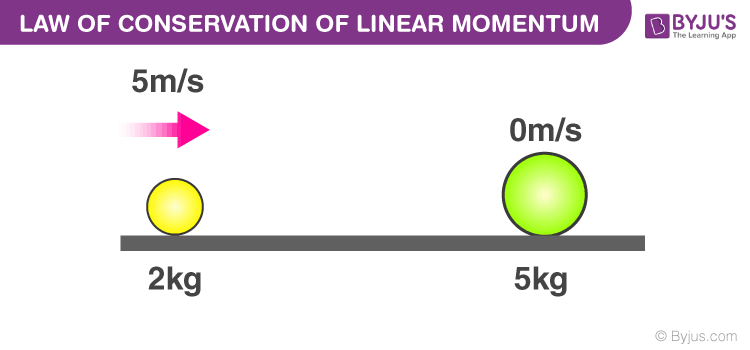# Law Of Conservation Of Linear Momentum

The linear momentum of a particle is defined as the product of the mass of the particle times the velocity of that particle. Conservation of momentum of a particle is a property exhibited by any particle where the total amount of momentum never changes. Linear momentum of a particle is a vector quantity and is denoted by $\underset{p}{\rightarrow}$

## Conservation of Linear Momentum

According to the conservation of linear momentum,

If the net external force acting on a system of bodies is zero, then the momentum of the system remains constant.

We have to remember that the momentum of the system is conserved and not that of the individual particles. The momentum of the individual bodies in the system might increase or decrease according to the situation, but the momentum of the system will always be conserved, as long as there is no external net force acting on it.

## Conservation of Linear Momentum Formula

The principle of conservation of momentum states that if two objects collide, then the total momentum before and after the collision will be the same if there is no external force acting on the colliding objects.

Conservation of linear momentum formula mathematically expresses the momentum of the system remains constant when the net external force is zero.
Initial momentum = Final momentum

 Pi = Pf

### Linear Momentum Formula

Linear momentum is mathematically expressed as:

$\overrightarrow{p}$ = m$\overrightarrow{v}$

$\overrightarrow{p}$ is the linear momentum

$\overrightarrow{v}$ is the linear velocity

m is the mass of the body## Conservation Of Linear Momentum Equation

The law of conservation of momentum can be explained from the second law of motion. Newton’s second law of motion says that the rate of change of linear momentum of a body is equal to the net external force applied to it.

Mathematically it is expressed as:

$\frac{dP}{dt} \\=\frac{(mv)}{dt} \\=m\frac{dv}{dt} \\=ma \\=F_{net}$

If the net external force acting on a body is zero, then the rate of change of momentum is also zero, which means that there is no change in momentum.

## Conservation Of Linear Momentum Example

Two bodies of mass M and m are moving in opposite directions with the velocities v. If they collide and move together after the collision, we have to find the velocity of the system.

Since there is no external force acting on the system of two bodies, momentum will be conserved.

Initial momentum = Final momentum

(Mv – mv) = (M+m)VFinal

From this equation, we can easily find the final velocity of the system.

## Conservation Of Linear Momentum Applications

One of the applications of conservation of momentum is the launching of rockets. The rocket fuel burns are pushed the exhaust gases downwards, and due to this, the rocket gets pushed upwards. Motorboats also work on the same principle, it pushes the water backward and gets pushed forwards in reaction to conserve momentum.

Here is a video that explains the application of PCLM using an example of a bomb explosion.Test your knowledge on Law of conservation of linear momentum

1. Vishnu choudhary

Good app

2. v g pillai

Well explained. Simple and to the point

3. Yasaswi

Nice app

4. Aayaan Thakur

excellent app, i would like to thank byju’s for providing such kinda help to students for their preparations.

5. good app

6. Manan Mitra

Awesome explanation and a very good app

7. Aishwarya Kshirsagar

Best app and best teachers ever had.

8. Azim Akhtar

Very good explanation, I am quite satisfied with it.

9. Dipak Sahoo

Excellent app

10. Sai charan

Conservation of linear momentum is equivalent to which laws of Newton?

1. Conservation of linear momentum is equivalent to Newton’s Third Law of Motion.

11. Hariharan

The example questions given are very useful

12. Explained very briefly!! Thank you for this content, it was really helpful.